8 Potential Energy and Conservation of Energy

8.1 Potential Energy of a System

Learning Objectives

By the end of this section, you will be able to:

• Relate the difference of potential energy to work done on a particle for a system without friction or air drag
• Explain the meaning of the zero of the potential energy function for a system
• Calculate and apply the gravitational potential energy for an object near Earth’s surface and the elastic potential energy of a mass-spring system

In Work, we saw that the work done on an object by the constant gravitational force, near the surface of Earth, over any displacement is a function only of the difference in the positions of the end-points of the displacement. This property allows us to define a different kind of energy for the system than its kinetic energy, which is called potential energy. We consider various properties and types of potential energy in the following subsections.

Potential Energy Basics

In Motion in Two and Three Dimensions, we analyzed the motion of a projectile, like kicking a football in (Figure). For this example, let’s ignore friction and air resistance. As the football rises, the work done by the gravitational force on the football is negative, because the ball’s displacement is positive vertically and the force due to gravity is negative vertically. We also noted that the ball slowed down until it reached its highest point in the motion, thereby decreasing the ball’s kinetic energy. This loss in kinetic energy translates to a gain in gravitational potential energy of the football-Earth system.

As the football falls toward Earth, the work done on the football is now positive, because the displacement and the gravitational force both point vertically downward. The ball also speeds up, which indicates an increase in kinetic energy. Therefore, energy is converted from gravitational potential energy back into kinetic energy.

Based on this scenario, we can define the difference of potential energy from point A to point B as the negative of the work done: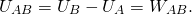This formula explicitly states a potential energy difference, not just an absolute potential energy. Therefore, we need to define potential energy at a given position in such a way as to state standard values of potential energy on their own, rather than potential energy differences. We do this by rewriting the potential energy function in terms of an arbitrary constant,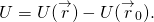The choice of the potential energy at a starting location of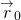is made out of convenience in the given problem. Most importantly, whatever choice is made should be stated and kept consistent throughout the given problem. There are some well-accepted choices of initial potential energy. For example, the lowest height in a problem is usually defined as zero potential energy, or if an object is in space, the farthest point away from the system is often defined as zero potential energy. Then, the potential energy, with respect to zero at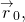is just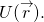As long as there is no friction or air resistance, the change in kinetic energy of the football equals the change in gravitational potential energy of the football. This can be generalized to any potential energy: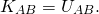Let’s look at a specific example, choosing zero potential energy for gravitational potential energy at convenient points.

Example

Basic Properties of Potential Energy

A particle moves along the x-axis under the action of a force given by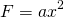, where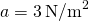. (a) What is the difference in its potential energy as it moves from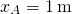to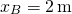? (b) What is the particle’s potential energy at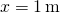with respect to a given 0.5 J of potential energy at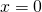?

Strategy

(a) The difference in potential energy is the negative of the work done, as defined by (Figure). The work is defined in the previous chapter as the dot product of the force with the distance. Since the particle is moving forward in the x-direction, the dot product simplifies to a multiplication (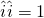). To find the total work done, we need to integrate the function between the given limits. After integration, we can state the work or the potential energy. (b) The potential energy function, with respect to zero at, is the indefinite integral encountered in part (a), with the constant of integration determined from (Figure). Then, we substitute the x-value into the function of potential energy to calculate the potential energy at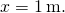Solution

1. The work done by the given force as the particle moves from coordinate x to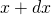in one dimension is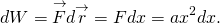Substituting this expression into (Figure), we obtain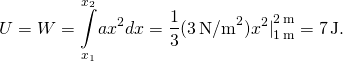2. The indefinite integral for the potential energy function in part (a) is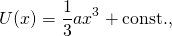and we want the constant to be determined by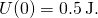Thus, the potential energy with respect to zero atis just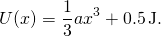Therefore, the potential energy atis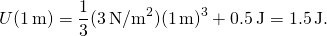Significance

In this one-dimensional example, any function we can integrate, independent of path, is conservative. Notice how we applied the definition of potential energy difference to determine the potential energy function with respect to zero at a chosen point. Also notice that the potential energy, as determined in part (b), atis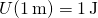and at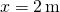is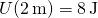; their difference is the result in part (a).

In (Figure), what are the potential energies of the particle atandwith respect to zero at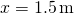? Verify that the difference of potential energy is still 7 J.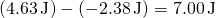Systems of Several Particles

In general, a system of interest could consist of several particles. The difference in the potential energy of the system is the negative of the work done by gravitational or elastic forces, which, as we will see in the next section, are conservative forces. The potential energy difference depends only on the initial and final positions of the particles, and on some parameters that characterize the interaction (like mass for gravity or the spring constant for a Hooke’s law force).

It is important to remember that potential energy is a property of the interactions between objects in a chosen system, and not just a property of each object. This is especially true for electric forces, although in the examples of potential energy we consider below, parts of the system are either so big (like Earth, compared to an object on its surface) or so small (like a massless spring), that the changes those parts undergo are negligible if included in the system.

Types of Potential Energy

For each type of interaction present in a system, you can label a corresponding type of potential energy. The total potential energy of the system is the sum of the potential energies of all the types. (This follows from the additive property of the dot product in the expression for the work done.) Let’s look at some specific examples of types of potential energy discussed in Work. First, we consider each of these forces when acting separately, and then when both act together.

Gravitational potential energy near Earth’s surface

The system of interest consists of our planet, Earth, and one or more particles near its surface (or bodies small enough to be considered as particles, compared to Earth). The gravitational force on each particle (or body) is just its weight mg near the surface of Earth, acting vertically down. According to Newton’s third law, each particle exerts a force on Earth of equal magnitude but in the opposite direction. Newton’s second law tells us that the magnitude of the acceleration produced by each of these forces on Earth is mg divided by Earth’s mass. Since the ratio of the mass of any ordinary object to the mass of Earth is vanishingly small, the motion of Earth can be completely neglected. Therefore, we consider this system to be a group of single-particle systems, subject to the uniform gravitational force of Earth.

In Work, the work done on a body by Earth’s uniform gravitational force, near its surface, depended on the mass of the body, the acceleration due to gravity, and the difference in height the body traversed, as given by (Figure). By definition, this work is the negative of the difference in the gravitational potential energy, so that difference is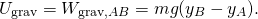You can see from this that the gravitational potential energy function, near Earth’s surface, is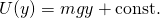You can choose the value of the constant, as described in the discussion of (Figure); however, for solving most problems, the most convenient constant to choose is zero for when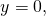which is the lowest vertical position in the problem.Don’t jump—you have so much potential (gravitational potential energy, that is). (credit: Andy Spearing)

Example

Gravitational Potential Energy of a Hiker

The summit of Great Blue Hill in Milton, MA, is 147 m above its base and has an elevation above sea level of 195 m ((Figure)). (Its Native American name, Massachusett, was adopted by settlers for naming the Bay Colony and state near its location.) A 75-kg hiker ascends from the base to the summit. What is the gravitational potential energy of the hiker-Earth system with respect to zero gravitational potential energy at base height, when the hiker is (a) at the base of the hill, (b) at the summit, and (c) at sea level, afterward?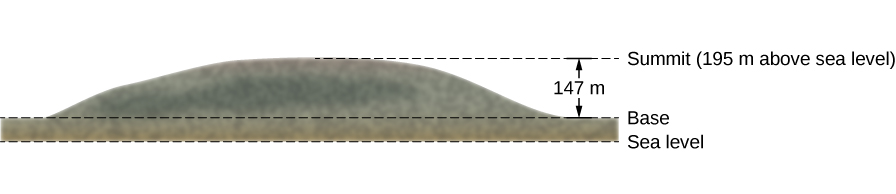Figure 8.3 Sketch of the profile of Great Blue Hill, Milton, MA. The altitudes of the three levels are indicated.

Strategy

First, we need to pick an origin for the y-axis and then determine the value of the constant that makes the potential energy zero at the height of the base. Then, we can determine the potential energies from (Figure), based on the relationship between the zero potential energy height and the height at which the hiker is located.

Solution

1. Let’s choose the origin for the y-axis at base height, where we also want the zero of potential energy to be. This choice makes the constant equal to zero and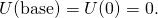2. At the summit,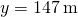, so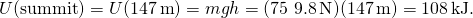3. At sea level,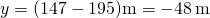, so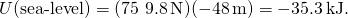Significance

Besides illustrating the use of (Figure) and (Figure), the values of gravitational potential energy we found are reasonable. The gravitational potential energy is higher at the summit than at the base, and lower at sea level than at the base. Gravity does work on you on your way up, too! It does negative work and not quite as much (in magnitude), as your muscles do. But it certainly does work. Similarly, your muscles do work on your way down, as negative work. The numerical values of the potential energies depend on the choice of zero of potential energy, but the physically meaningful differences of potential energy do not. [Note that since (Figure) is a difference, the numerical values do not depend on the origin of coordinates.]

Check Your Understanding What are the values of the gravitational potential energy of the hiker at the base, summit, and sea level, with respect to a sea-level zero of potential energy?

35.3 kJ, 143 kJ, 0

Elastic potential energy

In Work, we saw that the work done by a perfectly elastic spring, in one dimension, depends only on the spring constant and the squares of the displacements from the unstretched position, as given in (Figure). This work involves only the properties of a Hooke’s law interaction and not the properties of real springs and whatever objects are attached to them. Therefore, we can define the difference of elastic potential energy for a spring force as the negative of the work done by the spring force in this equation, before we consider systems that embody this type of force. Thus,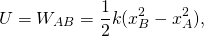where the object travels from point A to point B. The potential energy function corresponding to this difference is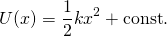If the spring force is the only force acting, it is simplest to take the zero of potential energy at, when the spring is at its unstretched length. Then, the constant is (Figure) is zero. (Other choices may be more convenient if other forces are acting.)

Example

Spring Potential Energy

A system contains a perfectly elastic spring, with an unstretched length of 20 cm and a spring constant of 4 N/cm. (a) How much elastic potential energy does the spring contribute when its length is 23 cm? (b) How much more potential energy does it contribute if its length increases to 26 cm?

Strategy

When the spring is at its unstretched length, it contributes nothing to the potential energy of the system, so we can use (Figure) with the constant equal to zero. The value of x is the length minus the unstretched length. When the spring is expanded, the spring’s displacement or difference between its relaxed length and stretched length should be used for the x-value in calculating the potential energy of the spring.

Solution

1. The displacement of the spring is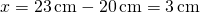, so the contributed potential energy is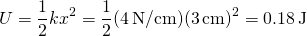.

2. When the spring’s displacement is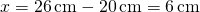, the potential energy is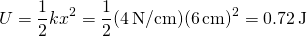, which is a 0.54-J increase over the amount in part (a).

Significance

Calculating the elastic potential energy and potential energy differences from (Figure) involves solving for the potential energies based on the given lengths of the spring. Since U depends on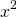, the potential energy for a compression (negative x) is the same as for an extension of equal magnitude.

When the length of the spring in (Figure) changes from an initial value of 22.0 cm to a final value, the elastic potential energy it contributes changes by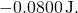Find the final length.

22.8 cm. Using 0.02 m for the initial displacement of the spring (see above), we calculate the final displacement of the spring to be 0.028 m; therefore the length of the spring is the unstretched length plus the displacement, or 22.8 cm.

Gravitational and elastic potential energy

A simple system embodying both gravitational and elastic types of potential energy is a one-dimensional, vertical mass-spring system. This consists of a massive particle (or block), hung from one end of a perfectly elastic, massless spring, the other end of which is fixed, as illustrated in (Figure).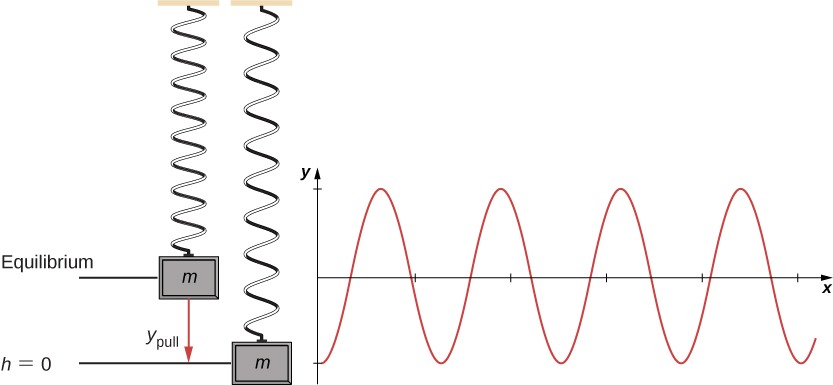Figure 8.4 A vertical mass-spring system, with the y-axis pointing upwards. The mass is initially at an equilibrium position and pulled downward to     An oscillation begins, centered at the equilibrium position.

First, let’s consider the potential energy of the system. Assuming the spring is massless, the system of the block and Earth gains and loses potential energy. We need to define the constant in the potential energy function of (Figure). Often, the ground is a suitable choice for when the gravitational potential energy is zero; however, in this case, the lowest point or when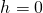is a convenient location for zero gravitational potential energy. Note that this choice is arbitrary, and the problem can be solved correctly even if another choice is picked.

We must also define the elastic potential energy of the system and the corresponding constant, as detailed in (Figure). The equilibrium location is the most suitable mathematically to choose for where the potential energy of the spring is zero.

Therefore, based on this convention, each potential energy and kinetic energy can be written out for three critical points of the system: (1) the lowest pulled point, (2) the equilibrium position of the spring, and (3) the highest point achieved. We note that the total energy of the system is conserved, so any total energy in this chart could be matched up to solve for an unknown quantity. The results are shown in (Figure).

Components of Energy in a Vertical Mass-Spring System
Gravitational P.E. Elastic P.E. Kinetic E.
(3) Highest Point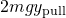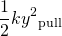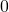(2) Equilibrium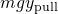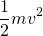(1) Lowest Point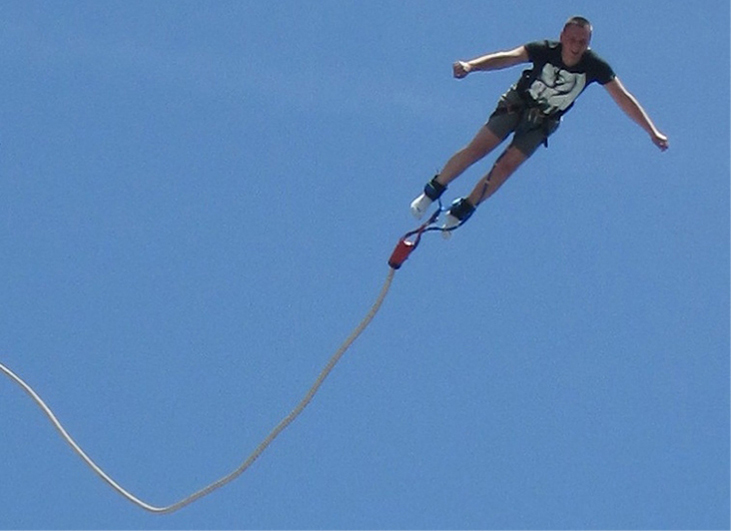Figure 8.5 A bungee jumper transforms gravitational potential energy at the start of the jump into elastic potential energy at the bottom of the jump.

Example

Potential Energy of a Vertical Mass-Spring System

A block weighing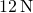is hung from a spring with a spring constant of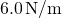, as shown in (Figure). The block is pulled down an additional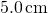from its equilibrium position and released. (a) What is the difference in just the spring potential energy, from an initial equilibrium position to its pulled-down position? (b) What is the difference in just the gravitational potential energy from its initial equilibrium position to its pulled-down position? (c) What is the kinetic energy of the block as it passes through the equilibrium position from its pulled-down position?

Strategy

In parts (a) and (b), we want to find a difference in potential energy, so we can use (Figure) and (Figure), respectively. Each of these expressions takes into consideration the change in the energy relative to another position, further emphasizing that potential energy is calculated with a reference or second point in mind. By choosing the conventions of the lowest point in the diagram where the gravitational potential energy is zero and the equilibrium position of the spring where the elastic potential energy is zero, these differences in energies can now be calculated. In part (c), we take a look at the differences between the two potential energies. The difference between the two results in kinetic energy, since there is no friction or drag in this system that can take energy from the system.

Solution

1. Since the gravitational potential energy is zero at the lowest point, the change in gravitational potential energy is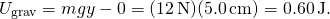2. The equilibrium position of the spring is defined as zero potential energy. Therefore, the change in elastic potential energy is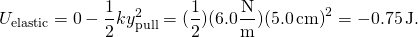3. The block started off being pulled downward with a relative potential energy ofThe gravitational potential energy required to rise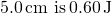. The energy remaining at this equilibrium position must be kinetic energy. We can solve for this gain in kinetic energy from (Figure),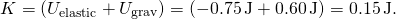Significance

Even though the potential energies are relative to a chosen zero location, the solutions to this problem would be the same if the zero energy points were chosen at different locations.

Suppose the mass in (Figure) is in equilibrium, and you pull it down another 3.0 cm, making the pulled-down distance a total of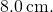The elastic potential energy of the spring increases, because you’re stretching it more, but the gravitational potential energy of the mass decreases, because you’re lowering it. Does the total potential energy increase, decrease, or remain the same?

It increases because you had to exert a downward force, doing positive work, to pull the mass down, and that’s equal to the change in the total potential energy.

View this simulation to learn about conservation of energy with a skater! Build tracks, ramps and jumps for the skater and view the kinetic energy, potential energy and friction as he moves. You can also take the skater to different planets or even space!

A sample chart of a variety of energies is shown in (Figure) to give you an idea about typical energy values associated with certain events. Some of these are calculated using kinetic energy, whereas others are calculated by using quantities found in a form of potential energy that may not have been discussed at this point.

Energy of Various Objects and Phenomena
Object/phenomenon Energy in joules
Big Bang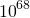Annual world energy use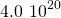Large fusion bomb (9 megaton)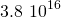Hiroshima-size fission bomb (10 kiloton)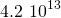1 barrel crude oil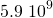1 ton TNT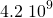1 gallon of gasoline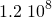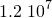1000-kg car at 90 km/h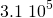Tennis ball at 100 km/h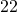Mosquito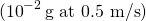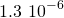Single electron in a TV tube beam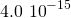Energy to break one DNA strand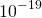Summary

• For a single-particle system, the difference of potential energy is the opposite of the work done by the forces acting on the particle as it moves from one position to another.
• Since only differences of potential energy are physically meaningful, the zero of the potential energy function can be chosen at a convenient location.
• The potential energies for Earth’s constant gravity, near its surface, and for a Hooke’s law force are linear and quadratic functions of position, respectively.

Conceptual Questions

The kinetic energy of a system must always be positive or zero. Explain whether this is true for the potential energy of a system.

The potential energy of a system can be negative because its value is relative to a defined point.

The force exerted by a diving board is conservative, provided the internal friction is negligible. Assuming friction is negligible, describe changes in the potential energy of a diving board as a swimmer drives from it, starting just before the swimmer steps on the board until just after his feet leave it.

Describe the gravitational potential energy transfers and transformations for a javelin, starting from the point at which an athlete picks up the javelin and ending when the javelin is stuck into the ground after being thrown.

If the reference point of the ground is zero gravitational potential energy, the javelin first increases its gravitational potential energy, followed by a decrease in its gravitational potential energy as it is thrown until it hits the ground. The overall change in gravitational potential energy of the javelin is zero unless the center of mass of the javelin is lower than from where it is initially thrown, and therefore would have slightly less gravitational potential energy.

A couple of soccer balls of equal mass are kicked off the ground at the same speed but at different angles. Soccer ball A is kicked off at an angle slightly above the horizontal, whereas ball B is kicked slightly below the vertical. How do each of the following compare for ball A and ball B? (a) The initial kinetic energy and (b) the change in gravitational potential energy from the ground to the highest point? If the energy in part (a) differs from part (b), explain why there is a difference between the two energies.

What is the dominant factor that affects the speed of an object that started from rest down a frictionless incline if the only work done on the object is from gravitational forces?

the vertical height from the ground to the object

Two people observe a leaf falling from a tree. One person is standing on a ladder and the other is on the ground. If each person were to compare the energy of the leaf observed, would each person find the following to be the same or different for the leaf, from the point where it falls off the tree to when it hits the ground: (a) the kinetic energy of the leaf; (b) the change in gravitational potential energy; (c) the final gravitational potential energy?

Problems

Using values from (Figure), how many DNA molecules could be broken by the energy carried by a single electron in the beam of an old-fashioned TV tube? (These electrons were not dangerous in themselves, but they did create dangerous X-rays. Later-model tube TVs had shielding that absorbed X-rays before they escaped and exposed viewers.)

40,000

If the energy in fusion bombs were used to supply the energy needs of the world, how many of the 9-megaton variety would be needed for a year’s supply of energy (using data from (Figure))?

A camera weighing 10 N falls from a small drone hovering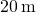overhead and enters free fall. What is the gravitational potential energy change of the camera from the drone to the ground if you take a reference point of (a) the ground being zero gravitational potential energy? (b) The drone being zero gravitational potential energy? What is the gravitational potential energy of the camera (c) before it falls from the drone and (d) after the camera lands on the ground if the reference point of zero gravitational potential energy is taken to be a second person looking out of a building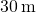from the ground?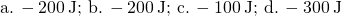Someone drops a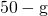pebble off of a docked cruise ship,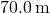from the water line. A person on a dock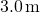from the water line holds out a net to catch the pebble. (a) How much work is done on the pebble by gravity during the drop? (b) What is the change in the gravitational potential energy during the drop? If the gravitational potential energy is zero at the water line, what is the gravitational potential energy (c) when the pebble is dropped? (d) When it reaches the net? What if the gravitational potential energy was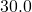Joules at water level? (e) Find the answers to the same questions in (c) and (d).

A cat’s crinkle ball toy of mass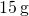is thrown straight up with an initial speed of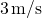. Assume in this problem that air drag is negligible. (a) What is the kinetic energy of the ball as it leaves the hand? (b) How much work is done by the gravitational force during the ball’s rise to its peak? (c) What is the change in the gravitational potential energy of the ball during the rise to its peak? (d) If the gravitational potential energy is taken to be zero at the point where it leaves your hand, what is the gravitational potential energy when it reaches the maximum height? (e) What if the gravitational potential energy is taken to be zero at the maximum height the ball reaches, what would the gravitational potential energy be when it leaves the hand? (f) What is the maximum height the ball reaches?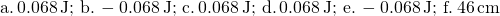Glossary

potential energy
function of position, energy possessed by an object relative to the system considered
potential energy difference
negative of the work done acting between two points in space